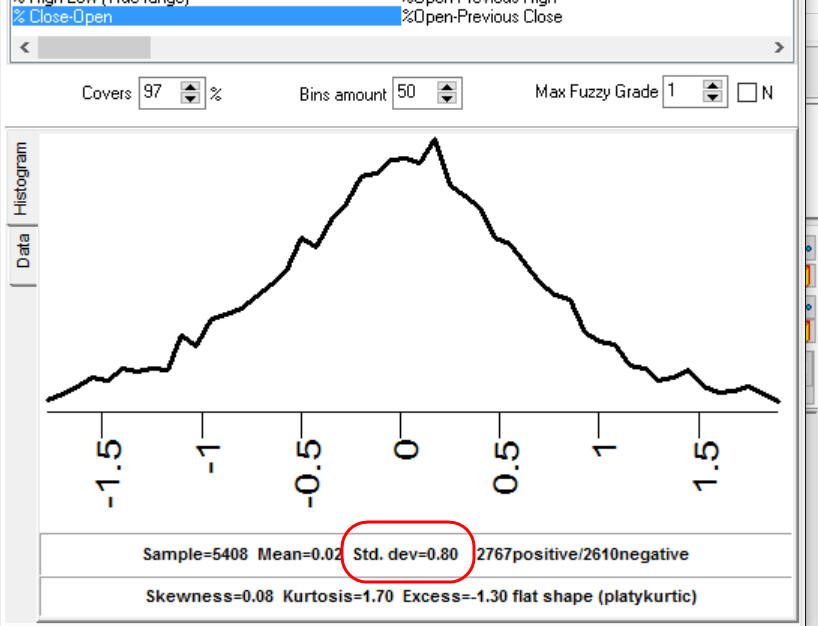"Fat tails" in finance

"Fat tails" is a statistical phenomenon,it appears in financial data tas well as inother fieldsthat involve human activity.

You should understand that this phenomenon has no mathematical solution, i.e. we cannot eliminate this phenomenon using some smart or very smart algorithms. Take it as "Memento mori" - the fact we have to accept whetherwe like it or not. This is the environment we live in.

Briefly "fat tails" phenomena may be formed this way: the power of accidental factor (or we think that this factor is accidental) ismuch more higherthan your common sense says you.

Here are some examples:

#1 impossible big drop: I would like to start with a classical example, example that is responsible (as I understand) for the name "fat tails".

Let's imagine that it is not the year 2016 now, but the year 1987. on October 19, 1987 S&P500 dropped more than 20%,  the largest one-day percentage decline.  The last drop in 1929 was much more smoother: though within a month Dow lost 45%, it was a gradually distributed decline (the biggest drop of 12.82% has occurred on October 28).

We ask ourselves: how often this huge drop may happen in the future, from statistical point of view?

I tell you how I would think then, in 1987, being a young scientist recently graduated from my University. From my point of view, it would be an elementary task, an easy one: we need to calculate a standard deviation for the percentage of one-day price change. Here it is, 0.8%Then we need to calculate sigma for this drop. The sigma for 20% price drop is: sigma=20%/0.8%=25. Ooops!

25 is a huge value of sigma. For a recent graduate studied the classical statistics, it says that 20% drop is practically impossible. There is simply no tables for sigma's value as 25. The biggest sigma in the tables I have is 6, and from the point of view of the classical statistics it means that 4.8% drop happens once in 4,000,000 years.

From the point of view of a trader, the situation looks differently: he/she has seen 4.8%+ drops before (1946, 1962). It means that something is wrong with our calculations. Technically it means that we cannot apply standard statistical formulas that were used to calculate that probability. Standard formulas are based on normal distribution and they underestimate big changes of the price movement, This is why they call it "fat tails" effect: the probability of big changes is much more higher than the normal distribution suggests. It means that "tails" of histogram are more bold than  in normal distribution:This subject is explained here: http://www.timingsolution.com/TS/Articles/cds/index.htm

#2 impossible big win/loss ratio: This is a model calculated with Timing Solution software. The Efficiency test shows us the information regarding trades around these vertical lines; we make 14 long winning trades versus 2 loss trades, 87% winning trades:We could create similar vertical lines that go right to the future, as far as we need. The question is: Can we trust these results? In other words, can we prolong this model into the future and trade in�the future with 87% accuracy?

Let's analyze this phenomenon from the point of view of the normal distributed statistics which is based on one simple idea: we dice a coin; if it is a head - I win; if tail - I lose. I diced that coin 11 times, and I win 10 times losing just one time. Would you like to use this coin for your play? Definitely yes, this coin has provided much more winning cases for me, you would expect that it will work the same way for you, why not?. But... Common sense tells us thatsomething is wrong with that coin, it likes "heads" more than "tails". This is not an occasional fact; the probability that it is not some chance is 100x(1-1/2^9)=99.8%.

Now let's go back to our stock market example. Can we use the above strategy to trade using our own trading account? Would you put your money on it? Your common sense should tell you: there is some pitfall there. And your common sense is right: in our example we analyze the price chart since 2012 when the market was in a very strong trend.

If we extend the analyzed period to cover uptrend and downtrend zones, our results become not so impressive - 32 ups versus 24 downs:You see, in these two examples we tried to apply standard statistical formula to estimate financial parameters and in both cases we failed in our estimations. This is why I wrote this class - be careful when somebody uses standard statistical formula.

One more example: somebody has invented a trading system that makes 100 trades, and 70 of them are winning trades. With the probability of 99.5%, this is not an occasional fact. However, it does not mean that the next 100 trades will be so good.